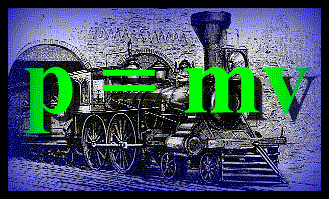# The Physics Department - Mechanics, MomentumObjects in motion are said to have a momentum. This momentum is a vector. It has a size and a direction. The size of the momentum is equal to the mass of the object multiplied by the size of the object's velocity. The direction of the momentum is the same as the direction of the object's velocity.

Momentum is a conserved quantity in physics. This means that if you have several objects in a system, most likely interacting with each other, but not being influenced by forces from outside of the system, then the total momentum of the system does not change over time. However, the momentum of each separate object within the system may change due to interactions such as collisions between objects. One object might change momentum, say losing some momentum, as another object changes momentum in an opposite manner by gaining the momentum lost by the first, and so the total momentum in the system stays constant. Momentum is conserved.

Custom Search